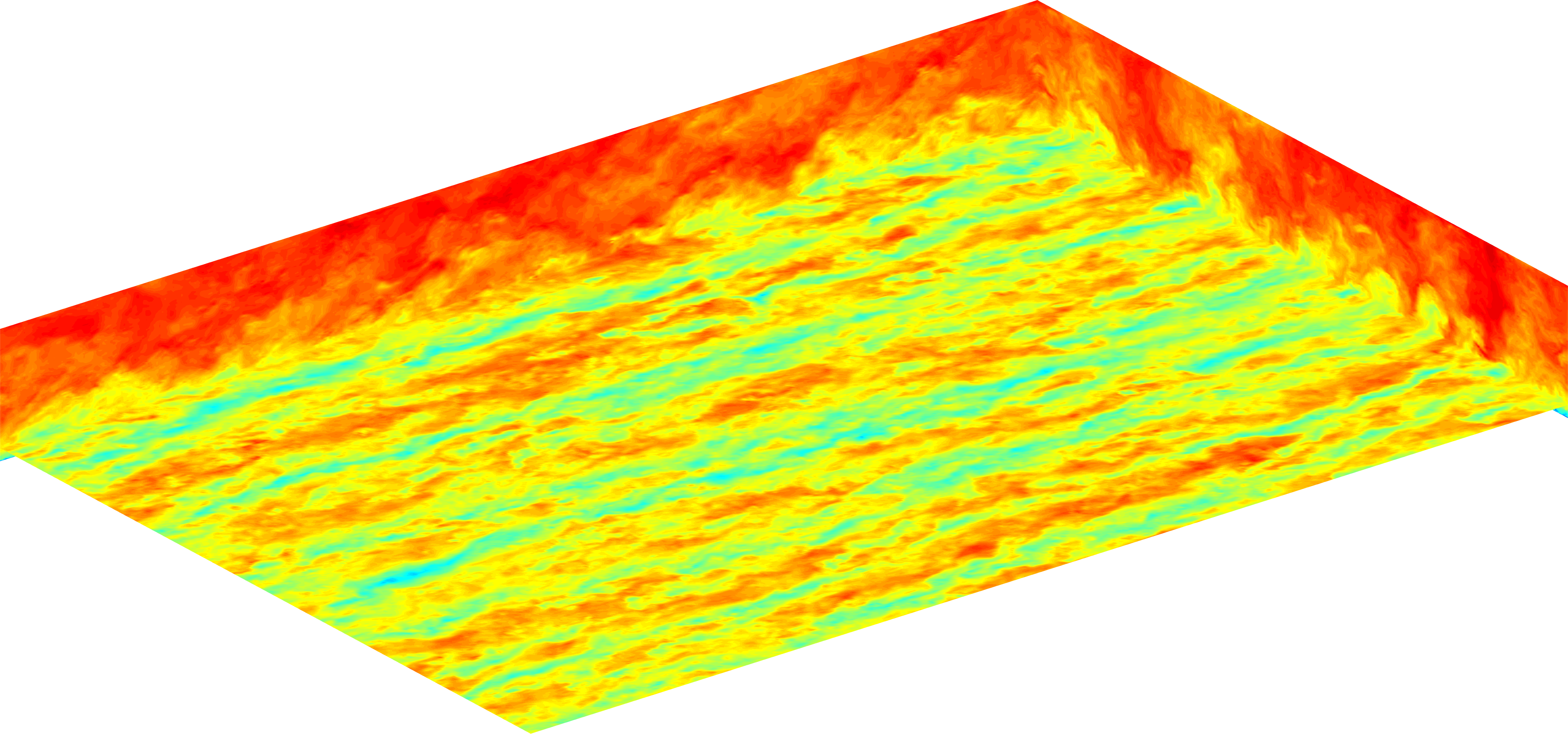# LESGOLESGO is a parallel pseudo-spectral large-eddy simulation code.

# Wall models

Several options are available for the top and bottom boundary conditions:

• The stress free condition sets the stresses and vertical derivatives of the velocity equal to zero $\tau_{xz} = \tau_{yz} = 0 \qquad \qquad \frac{\partial u}{\partial z} = \frac{\partial v}{\partial z} = 0.$

• The viscous wall condition uses molecular viscosity and calculates the wall stress using the values at the first grid point away from the wall.

• The equilibrium wall model applies the local law-of-the-wall expression for the wall stress $\tau_w = -\left[ \frac{\kappa}{\ln\left(z/z_0\right)}\right]^2 \left( \tilde{u}^2 + \tilde{v}^2\right)$ where $$\kappa$$ is the von Kármán constant, $$z_0$$ is the surface roughness height, and velocities have been test filtered at scale $$2\Delta$$.

• The integral wall model uses a vertical profile similar to the classical integral method of von Kármán and Pohlhausen.

Yang X, Sadique J, Mittal R, Meneveau C. “Integral wall model for large eddy simulations of wall-bounded turbulent flows.” Physics of Fluids 27 (2015). 025112.

Bou-Zeid E, Meneveau C, Parlange MB. “A scale-dependent Lagrangian dynamic model for large eddy simulation of complex turbulent flows.” Physics of Fluids 17 (2005). 025105.